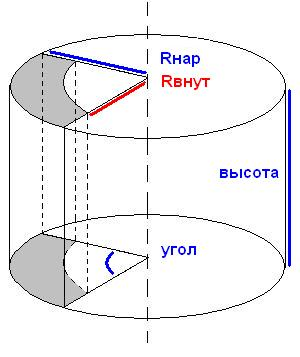homechevron_rightProfessionalchevron_rightEngineering

# Volume of the cylinder segment

Calculates volume of the cylinder segment

Our user asked to make a cylinder segment volume calculator. I imagine the segment like this - see pictureAccordingly, we set four parameters and obtain the volume segment. If the inner radius is set 0 and the number of degrees is 360, we will calculate the volume of cylinder and so on.

The formula is simple - the area difference of the segments obtained by the first and the second radius, multiplied by the height.
Segment area#### Volume of the cylinder segment

Digits after the decimal point: 2
Segment volume

URL copied to clipboardPLANETCALC, Volume of the cylinder segment